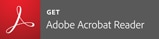# Trace Quantity Measurement System - Applications

Applications Creation Date

2018-06-01## Sample Analysis

### Quantitation of Trace Quantities of Nucleic Acids

Samples of nucleic acids and proteins are often only available in small quantities. Accordingly, cells capable of trace quantity measurements are required. In this example, trace quantity measurements of nucleic acids were performed using a TrayCell™ with an optical path length of 1.0 mm, which allows measurements with sample volumes of 3 to 5 μL.

Lambda-DNA, a type of double stranded DNA often utilized in nucleic acid analysis, was analyzed.

A 1.0 Abs concentration sample was measured repeatedly 10 times with good precision, as follows:
The standard deviation was 0.014629 with a CV of 1.34 %.
The calibration curve formula was Y = 0.00201x + 0.00827, and the correlation coefficient was r2 = 0.99989.

Overlaid Spectra for Nucleic Acids with Different Concentrations